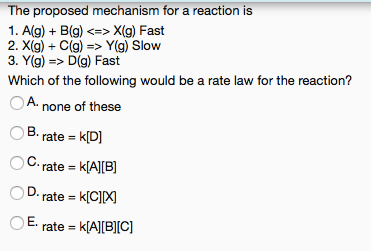# The proposed mechanism for a reaction is 1. A(g) + B(g) <=> X(g) Fast 2. X(g) + C(g) => Y(g) Slow 3. Y(g) => D(g) Fast Which of the following would be a rate law for the reaction? a) none of these b) rate = k[D] c) rate = k[A][B] d) rate = k[C][X] e) rate = k[A][B][C]